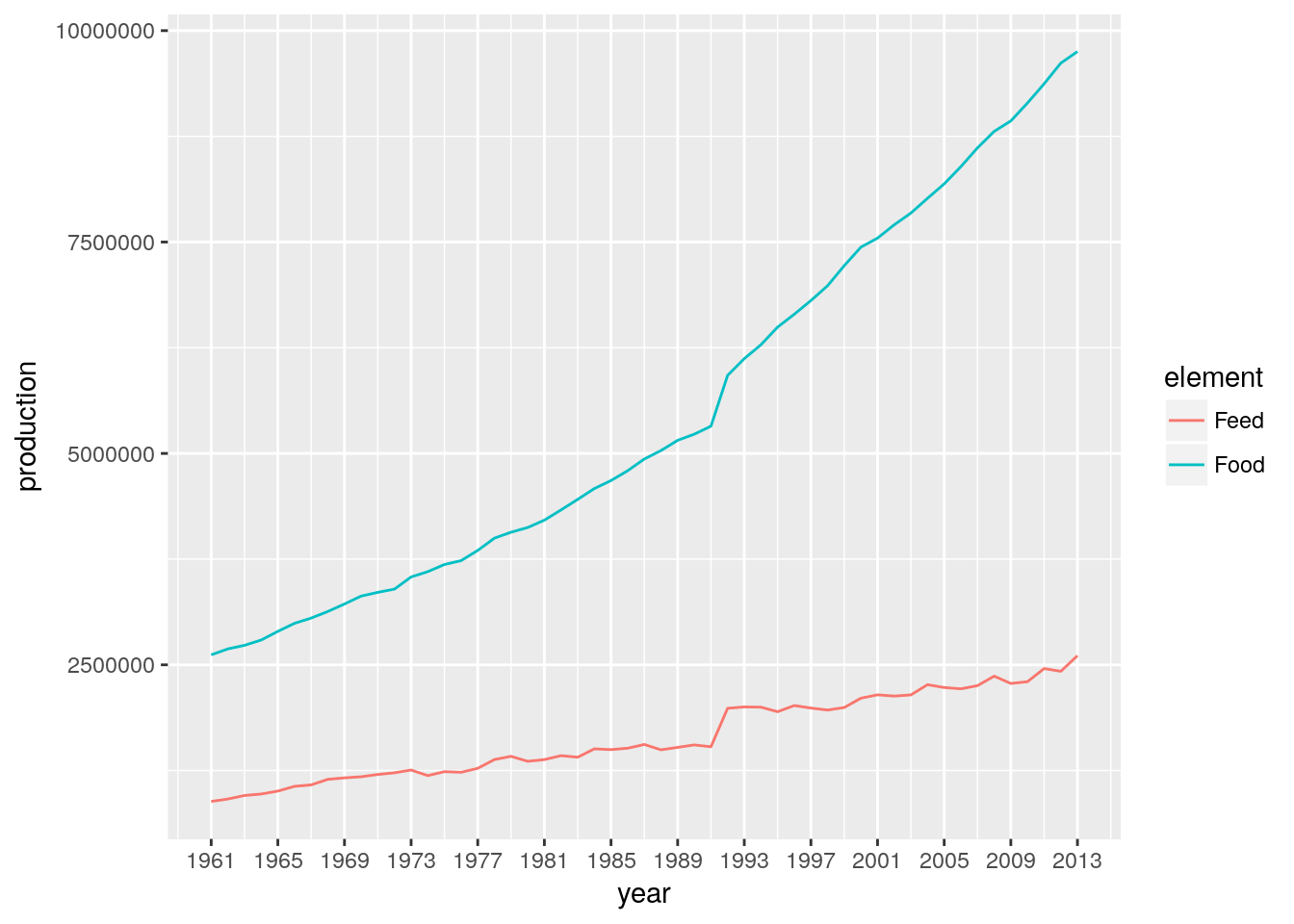## Setup

``````library(tidyverse)
library(sqldf)
library(visdat)
library(broom)
library(lmtest)
library(forecast)
library(hts)``````

Load the feed data and change the column names to lower-case with underscores.

``````feed_raw <- "feed.csv" %>%
rename_all(.funs = function(x) {gsub(" ", "_", x) %>% tolower})``````
``````## Parsed with column specification:
## cols(
##   .default = col_integer(),
##   `Area Abbreviation` = col_character(),
##   Area = col_character(),
##   Item = col_character(),
##   Element = col_character(),
##   Unit = col_character(),
##   latitude = col_double(),
##   longitude = col_double()
## )``````
``## See spec(...) for full column specifications.``

At this point we’ve loaded the raw csv data with the `read_csv` function. `read_csv` does not convert strings to factors, but we do have a few factors here. The year variables are all integers and begin with Y, while the longitude and latitude variables are numerics. The rest we convert to factors.

``````feed_raw <- feed_raw %>% mutate_at(
vars(-starts_with("y"), -starts_with("l")),
funs(as.factor)
)``````

The initial wide format isn’t very convenient for modelling, but it is appropriate for visualising the missing data. Some missing data, but it seems that a row is either complete, or is missing an entire string of years. It actually looks as though collection starts in different years for each item, but once collection starts it continues uninterrupted. What happened in 1993?

`````````r
feed_raw %>% vis_dat(warn_large_data = FALSE) +
theme(axis.text = element_text(size = 8))
```

<img src="EDA_files/figure-html/unnamed-chunk-2-1.png" width="100%" />``````

Let’s clean this up. We gather all of those year columns into a simple Key/Value long format. We then take the four right-most characters of each year, effectively dropping the “Y” in front of each year.

``````feed <- feed_raw %>%
gather(key = year, value = production, starts_with("y")) %>%
mutate(year = year %>% substr(2, 5) %>% as.numeric)``````

Change in food and feed production over the years.

``````feed %>%
group_by(year, element) %>%
summarise(production = production %>% sum(na.rm = TRUE)) %>%
ggplot(aes(x = year, y = production, group = element, colour = element)) +
geom_line() +
scale_x_continuous(
breaks = seq(min(feed\$year), max(feed\$year), 4)
)``````We use an SQL join to introduce a change column for each (Area, Item, Element) tuple, so that we can track how much production has increased or decreased since last year. I can’t think of a clean way to do this with the usual R `merge` function, and SQL seems so well-suited for the task.

``````feed <- sqldf(
"
SELECT       CURRENTYEAR.*
,LASTYEAR.production AS production_last_year
,CURRENTYEAR.production - LASTYEAR.production as production_change
FROM        feed AS CURRENTYEAR
LEFT JOIN   feed AS LASTYEAR
ON      CURRENTYEAR.area = LASTYEAR.area
AND     CURRENTYEAR.item = LASTYEAR.item
AND     CURRENTYEAR.element = LASTYEAR.element
AND     CURRENTYEAR.year = LASTYEAR.year + 1
"
)``````

Heat map showing change in production for each category.

``````feed %>%
group_by(year, item) %>%
filter(!is.na(production_last_year)) %>%
summarise(
production = production %>% sum(na.rm = TRUE),
production_last_year = production_last_year %>% sum(na.rm = TRUE)
) %>%
mutate(percent_production_change =
(production - production_last_year) / production_last_year) %>%
ggplot(aes(x = year, y = item, fill = percent_production_change)) +
geom_raster() +
scale_fill_gradient2(low = "blue", mid = "white", high = "red",
limits = c(-0.5, 0.5))``````